# THE ZOOM FFT

The Zoom FFT is interesting because it blends complex downconversion, lowpass filtering, and sample rate change through decimation in a spectrum analysis application. The Zoom FFT method of spectrum analysis is used when fine spectral resolution is needed within a small portion of a signal's overall frequency range. This technique is more efficient than the traditional FFT in such a situation.

Think of the spectral analysis situation where we require fine frequency resolution, closely spaced FFT bins, over the frequency range occupied by the signal of interest shown in Figure 13-52(a). (The other signals are of no interest to us.) We could collect many time samples and perform a large-size radix-2 FFT to satisfy our fine spectral resolution requirement. This solution is inefficient because we'd be discarding most of our FFT results. The Zoom FFT can help us improve our computational efficiency through:

• frequency translation by means of complex downconversion,
• low-pass filtering,
• decimation, and finally
• performing a smaller size FFT.

Figure 13-52. Zoom FFT spectra: (a) input spectrum; (b) processing scheme; (c) downconverted spectrum; (d) filtered and decimated spectrum.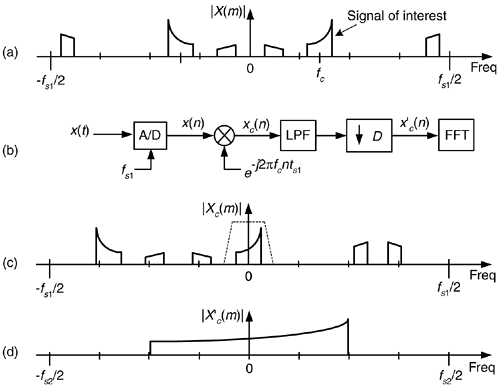The process begins with the continuous x(t) signal being digitized at a sample rate of fs1 by an analog-to-digital (A/D) converter yielding the N-point x(n) time sequence whose spectral magnitude is |X(m)| in Figure 13-52(a). The Zoom FFT technique requires narrowband filtering and decimation in order to reduce the number of time samples prior to the final FFT, as shown in Figure 13-52(b). The downconverted signal's spectrum, centered at zero Hz, is the |Xc(m)| shown in Figure 13-52(c). (The lowpass filter's frequency response is the dashed curve.) After lowpass filtering xc(n), the filter's output is decimated by an integer factor D yielding a time sequencewhose sample rate is fs2 = fs1/D prior to the FFT operation. The key here is that the length ofis N/D, allowing a reduced-size FFT. (N/D must be an integer power of two to enable the use of radix-2 FFTs.) We perform the FFT only over the decimated signal's bandwidth. It's of interest to note that, because its input is complex, the N/D-point FFT has a non-redundant frequency analysis range from –fs2/2 to +fs2/2. (Unlike the case of real inputs, where the positive and negative frequency ranges are redundant.)

The implementation of the Zoom FFT is given in Figure 13-53, where all discrete sequences are real valued.

Figure 13-53. Zoom FFT processing details.Relating the discrete sequences in Figure 13-52(b) and Figure 13-53, the complex time sequence xc(n) is represented mathematically as: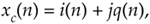while the complex decimated sequenceis

Equation 13-101The complex mixing sequence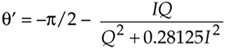, where ts1 = 1/fs1, can be represented in the two forms of

Equation 13-102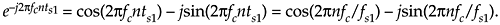Let's use an example to illustrate the Zoom FFT's ability to reduce standard FFT computational workloads. Say we have a signal sampled at fs1 = 1024 kHz, and we require 2 kHz FFT bin spacing for our spectrum analysis resolution. These conditions require us to collect 512 samples (1024 kHz/2 kHz) and perform a 512-point FFT. If the signal of interest's bandwidth is less than fs1/8, we could lowpass filter, use a decimation factor of D = 8, and only perform a 512/8 = 64-point FFT and still achieve the desired 2 kHz frequency resolution. We've reduced the 512-point FFT (requiring 2304 complex multiplies) to a 64-point FFT (requiring 192 complex multiplies). That's an computational workload reduction of more than 90%!

We're using the following definition for the percent computation reduction in complex multiplies afforded by the (N/D)-point Zoom FFT over the standard N-point FFT,

Equation 13-103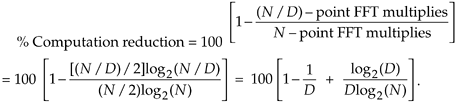Plotting Eq. (13-103) for various decimation factors over a range of FFT sizes (all integer powers of two) yields the percent of FFT computational reduction curves in Figure 13-54.

Figure 13-54. Percent computational workload reduction, in complex multiplies, of an (N/D)-point Zoom FFT relative to a standard N-point FFT.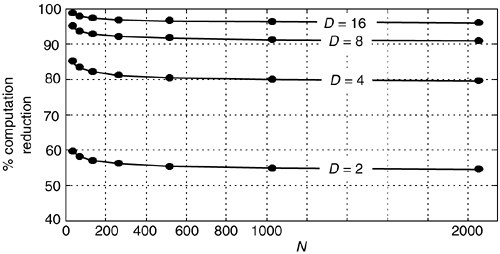Amazon